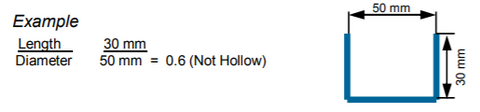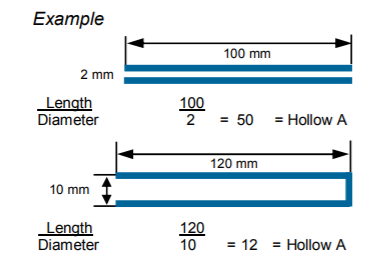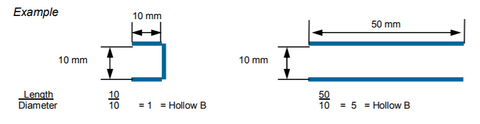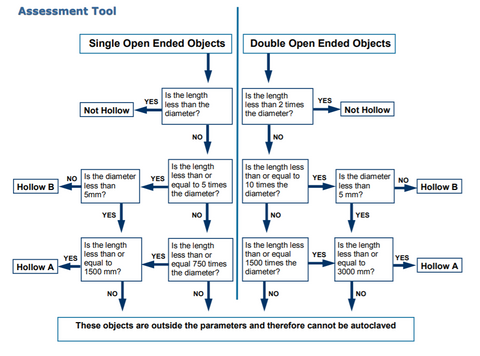# How to determine a hollow instrument?

To ensure that medical devices and instruments are properly sterilised, it is important to first determine their hollow classification so that the correct type of steriliser / steriliser cycle can be selected for the process.

Australian / New Zealand Standard AS/NZS 4815:2006 outlines the definitions for the different types of hollows.  These definitions are based on the definitions in the European Standard EN 13060. This sheet will reference both documents to ensure absolute clarity on the definition.

There are three definitions outlined in the standard.

1. Objects which are not hollow
2. Hollow A objects
3. Hollow B objects

#### 1. Objects which are not hollow

Some objects, although having similar characteristics, are not subjected to the classification of a hollow object.  In simple terms, objects are not hollow if the ratio of the length of the cavity to diameter is less than 1.

For example:#### 2. Hollow A objects

AS/NZS 4815:2006 “An object whose ratio of length of cavity to diameter is greater than 1 and greater than 5”

EN 13060:2004 “single ended open space where the ratio of length to diameter of the cavity is greater than or equal to 1 and less than/or equal to 750 (1 <  L/D < 750) and where the length of the cavity is not greater than 1500mm (L < 1500mm) and double ended open space where the ratio of length to diameter of the cavity is greater than/or equal to 2 and less than or equal to 1500 ( 2 < L/D < 1500) and where the length of the cavity is not greater than 3000mm (L < 3000mm) and which is not hollow load B.

Single Ended If the length is greater than or equal to 1 and up to 750 times the diameter and the length does not exceed 1500 mm and it is not Hollow B, then it is a Hollow A.

Double Ended If the length is greater than 2 and up to 1500 times the diameter and the length does not exceed 3000 mm and it is not Hollow B, then it is a Hollow A.Some examples of Hollow A objects may be handpieces, reusable aspirator tips and triple syringes.

#### 3. Hollow B objects

AS/NZS 4815:2006 “An object whose ratio of length of cavity to diameter is greater than 1 and less than 5”

EN 13060:2004 “single ended open space where the ratio of length to diameter of the cavity is greater than or equal to 1 and less than/or equal to 5 (1 <  L/D < 5) and where the diameter is greater than or equal to 5mm (D > 5mm) and double ended open space where the ratio of length to diameter of the cavity is greater than/or equal to 2 and less than or equal to 10 ( 2 < L/D < 10) and where the diameter is greater than or equal to 5mm (D > 5mm)

Single Ended If the length is greater than or equal to 1 and up to 5 times the diameter and the diameter is greater than or equal to 5mm then it is a Hollow B.

Double Ended If the length is greater than 2 and up to 10 times the diameter and the diameter is greater than or equal to 5mm then it is a Hollow B.#### Assessment ToolPlease note that this information is general in nature and is based on the Australian and European Standards listed above and should only be taken as a guide and not advice.